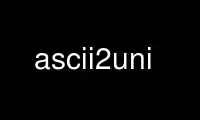# ascii2uni - Online in the CloudThis is the command ascii2uni that can be run in the OnWorks free hosting provider using one of our multiple free online workstations such as Ubuntu Online, Fedora Online, Windows online emulator or MAC OS online emulator

### PROGRAM:

#### NAME

ascii2uni - convert 7-bit ASCII representations to UTF-8 Unicode

#### SYNOPSIS

ascii2uni [options] (<input file name>)

#### DESCRIPTION

ascii2uni converts various 7-bit ASCII representations to UTF-8. It reads from the
standard input and writes to the standard output. The representations understood are
listed below under the command line options. If no format is specified, standard
hexadecimal format (e.g. 0x00e9) is assumed.

#### COMMANDLINEOPTIONS

-a <format> Convert from the specified format. Formats may be specified by means of the
following arbitrary single character codes, by means of names such as "SGML_decimal", and
by examples of the desired format.

A Convert hexadecimal numbers with prefix U in angle-brackets (<U00E9>).

B Convert \x-escaped hex (e.g. \x00E9)

C Convert \x escaped hexadecimal numbers in braces (e.g. \x{00E9}).

D Convert decimal HTML numeric character references (e.g. &#0233;)

E Convert hexadecimal with prefix U (U00E9).

F Convert hexadecimal with prefix u (u00E9).

G Convert hexadecimal in single quotes with prefix X (e.g. X'00E9').

H Convert hexadecimal HTML numeric character references (e.g. &#x00E9;)

I Convert hexadecimal UTF-8 with each byte's hex preceded by an =-sign (e.g.
=C3=A9) . This is the Quoted Printable format defined by RFC 2045.

J Convert hexadecimal UTF-8 with each byte's hex preceded by a %-sign (e.g.
%C3%A9). This is the URIescape format defined by RFC 2396.

K Convert octal UTF-8 with each byte escaped by a backslash (e.g. \303\251)

L Convert \U-escaped hex outside the BMP, \u-escaped hex within the BMP
(U+0000-U+FFFF).

M Convert hexadecimal SGML numeric character references (e.g. \#xE9;)

N Convert decimal SGML numeric character references (e.g. \#233;)

O Convert octal escapes for the three low bytes in big-endian order(e.g.
\000\000\351))

P Convert hexadecimal numbers with prefix U+ (e.g. U+00E9)

Q Convert HTML character entities (e.g. &eacute;).

R Convert raw hexadecimal numbers (e.g. 00E9)

S Convert hexadecimal escapes for the three low bytes in big-endian order (e.g.
\x00\x00\xE9)

T Convert decimal escapes for the three low bytes in big-endian order (e.g.
\d000\d000\d233)

U Convert \u-escaped hexadecimal numbers (e.g. \u00E9).

V Convert \u-escaped decimal numbers (e.g. \u00233).

X Convert standard hexadecimal numbers (e.g. 0x00E9).

Y Convert all three types of HTML escape: hexadecimal and decimal character
references and character entities.

0 Convert hexadecimal UTF-8 with each byte's hex enclosed within angle brackets
(e.g. <C3><A9>).

1 Convert Common Lisp format hexadecimal numbers (e.g. #x00E9).

2 Convert Perl format decimal numbers with prefix v (e.g. v233).

3 Convert hexadecimal numbers with prefix \$ (e.g. \$00E9).

4 Convert Postscript format hexadecimal numbers with prefix 16# (e.g. 16#00E9).

5 Convert Common Lisp format hexadecimal numbers with prefix #16r (e.g. #16r00E9).

6 Convert ADA format hexadecimal numbers with prefix 16# and suffix # (e.g.
16#00E9#).

7 Convert Apache log format hexadecimal UTF-8 with each byte's hex preceded by a
backslash-x (e.g. \xC3\xA9).

8 Convert Microsoft OOXML format hexadecimal numbers with prefix _x and suffix _
(e.g. _x00E9_).

9 Convert %\u-escaped hexadecimal numbers (e.g. %\u00E9).

-h Help. Print the usage message and exit.

-v Print program version information and exit.

-m Accept deprecated HTML entities lacking final semicolon, e.g. "&#x00E9" in place
of "&#x00E9;".

-p Pure. Assume that the input consists entirely of escapes except for arbitrary (but
non-null) amounts of separating whitespace.

-q Be quiet. Do not chat unnecessarily.

-Z <format>
Convert input using the supplied format. The format specified will be used as the
format string in a call to sscanf(3) with a single argument consisting of a pointer
to an unsigned long integer. For example, to obtain the same results as with the -U
flag, the format would be: \u%04X.

If the format is Quoted-Printable, although it is not strictly speaking conversion of an
ASCII escape to Unicode, in accordance with RFC 2045, if an equal-sign occurs at the end
of an input line, both the equal-sign and the immediately following newline are skipped.

All options that accept hexadecimal input recognize both upper- and lower-case hexadecimal
digits.

#### EXITSTATUS

The following values are returned on exit:

0 SUCCESS
The input was successfully converted.

3 INFO The user requested information such as the version number or usage synopsis and
this has been provided.

An incorrect option flag was given on the command line.

7 OUT OF MEMORY